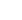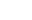(972) 865-6205info@privatecapitalinvestors.com

# How to Find ROI on Your Real Estate Investment

Whether you are a beginner in the real estate investing game or an old player, the first thing you will have to look is the value of ROI or Return on Investment.

It all comes down to – how much money am I going to make out of this deal?

At the end of the day in real estate investing or in any business for that matter. Knowing the Return on Investment helps you understand how profitable your real estate investing would be and how lucrative it can be in the long run.

Hence, you should know how to calculate Return On Investment on various properties for you to make the best decisions with your commercial real estate properties.

Above all, knowing how to calculate ROI helps you analyze what properties are the most lucrative ones. This makes it easy for you to compare various options and narrow down your options accordingly.

There are numerous ways of calculating ROI. The reason behind is that it’s not possible to determine the ROI of all properties using one simple formula as there are many variables to calculating ROI.

The formula of calculating ROI which may be used for one property, may not make sense to be applied for another property.

Thus, with numerous formulae to calculate ROI, all of which leading to different results, leaves investors confused about what formula to use in a given situation.

This blog outlines the most commonly applied ROI calculating methods and the situations where it makes sense to apply these formulae to determine your investment’s ROI.

Bottom line: There is no one single way or method of calculating ROI. Knowing a handful of them along with scenarios where it makes sense to use them is thus crucial. And that’s what we’re going to cover in this blog. Read on.

### How to calculate Return On Investment on real estate properties

There are three most widely used ways of calculating ROI on real estate properties:

1. The Basic Method
2. The Cost Method
3. The Out-of-pocket method

#### Number 1 – The Basic Method

The Basic Method, as the name suggests, is the simplest way of calculating ROI. In this method, Return on Investment is calculated by dividing the profit by the cost of the investment.

ROI under The Basic Method = (Gain from an investment – Cost of investment) / Cost of an investment.

For instance: If you purchase a property that costs you \$ 50,000 and the gain you make from such a purchase is \$ 80,000, then the ROI on this investment is 60% [Calculated (\$80,000 – \$50,000)/\$50,000].

This method is the easiest to apply, but it doesn’t provide the most reliable results.

The reason being – this method does not consider other related expenses which may have been occurred in the process of buying a property and thus, does not provide a clear picture of how much returns your investment can provide you.

This method gives only a bare idea of how much you can make from a real estate deal, but cannot be used to determine whether or not you should invest in a real estate property.

This method generally provides a figure that might be more attractive than the actual returns. Hence, this method using the go-to method for investors who want to base their decision of buying a property on the ROI arrived upon.

However, sometimes lending institutions who wish to sell their loans to realtors use this method to paint a colorful picture to the investor. You must be cautious with such transactions and must pay close attention to what method someone is using for arriving at the ROI as opposed to buying these rates at the face of it.

The next two methods explained below are more relevant and accurate, providing you more reliable results.

#### Number 2 – The Cost Method

The Cost Method of calculating ROI is suitable when you have purchased the property fully in cash. This method is also called as the “Capitalization Rate” method, and the ROI is referred to as the Cap Rate. So, if you are told that the Cap Rate of a property is 9%, it means that the ROI is 9% and that the method using is the Cost Method.

According to this method, the Return on Investment is calculated by dividing the Net Operating Income of the property by the purchase price.

ROI calculated according to the cost method = (Net Operating Income/Purchase price of the property) * 100

For instance: Let’s say you bought a real estate property at a purchase price of \$100,000 in cash. You decide to rent this property out at \$600 per month, making your annual rental income to be \$7200.

You further paid \$1200 as closing costs (the costs incurred while closing the purchase transaction like documentation or legal work or fees paid to real estate brokers etc.) and incurred an extra \$8800 for remodeling your property. Thus, the purchase price of your commercial real estate property is now \$110,000.

For deriving at the Net Operating Income, it is important to deduct all the expenses incurred in a year to earn the rental income every month.

In this instance, let’s say you pay \$1000 for property taxes, \$500 for maintenance, \$400 for insurance and \$100 on advertisement expenses you will incur to attract your prospective tenants.

So, the Net Operating Income is thus \$5200. Thus, the ROI = 4.73% [ calculated as (\$5200/\$110,000) * 100].

The Cost Method thus provides a more accurate picture on the return on investment calculated year on year.

Note that the ROI keeps changing based on the changing net operating income, which is influenced by the annual expenses you incur on your property as well as the varying monthly rental income rates when there is a change in the rental agreement or terms.

As an investor, remember to deduct all the expenses that are likely to occur in a year for arriving at most accurate results.

In most cases, the actual returns on real estate investing are quite similar to the speculated returns when The Cost Method is applied, and hence this approach is definitely a go-to approach for investors investing in real estate properties by paying full in cash.

#### Number 3 – The out-of-pocket Method

The Out-of-pocket method of calculating ROI makes the best sense to be used when the investment is made by taking out a mortgage. This method is also called as ‘Cash on Cash Return’ approach.

Under this method, the ROI of a property is determined by dividing the Net Operating Income by the total cash investment of the property.

ROI calculated using the Out-of-pocket method = (Net Operating Income/total cash investment of the property) * 100

For instance: Take the same example of \$100,000 purchase priced property stated above, but instead of financing it fully with cash, let’s assume you decide to take up a mortgage on the same.

The down payment costs of such loans is an average of 20%, so let’s say the down payment works out to be \$20,000. Let’s assume the closing costs to be same as \$2500 (the rise in the closing costs can be attributed to the higher costs incurred in case of a mortgage as there are more expenses related to raising a mortgage from private lenders or lending institutions) and the property remodeling costs to be the same as \$8800.

Thus, the total cash investment of the property now works out to be \$31,300 (calculated as \$20,000+\$2500+\$8800).

The next step is to calculate the Net Operating Income. After deducting all the annual expenses from the annual rental income, you should deduct the mortgage-related expenses like mortgage principal payments and the interest payments.

After having deducted all of these expenses put together, you’ll arrive at the Net Operating Income. Let’s assume the Net Operating Income to be \$3500. The ROI calculated now using the Out-of-pocket method would be 11.18% [calculated as (\$3500/\$31,300) * 100].

Why is there a difference in the ROI calculated for the same property under the two methods?

You might be wondering about the difference in the ROI calculation for the same property with a purchase price of \$100,000, where the out-of-pocket Method is providing a higher ROI as compared to the Cost Method.

Does that mean that the results are inaccurate? No. It simply means that the investors who buy out mortgages for investing in real estate investments have the leverage of such delayed out-of-pocket costs, leading to a higher ROI.

This is also one of the reasons why it’s a good idea to finance your investments with mortgages as opposed to making full payments in cash.

With various kinds of commercial real estate loans out there, choosing the best loans can thus greatly increase your overall Return on Investments. Choosing the right kind of commercial real estate lender who will provide you loans at best rates and terms is also very crucial, which can determine a great deal about your overall commercial real estate investing success!

## Asset-Backed (ABS) vs. Mortgage-Backed Securities (MBS)

As the market develops, various indices about the operation and rate of change in the assets are created. For example, asset-backed securities (ABS), a category of debt security, are backed by a collection of assets. The support can range from school loans to credit...